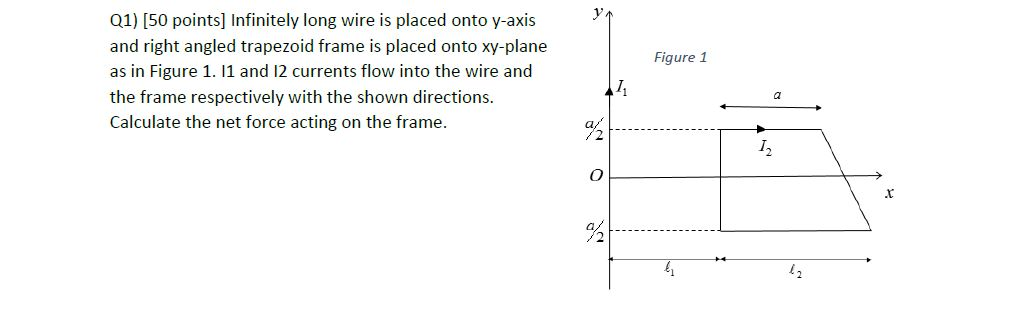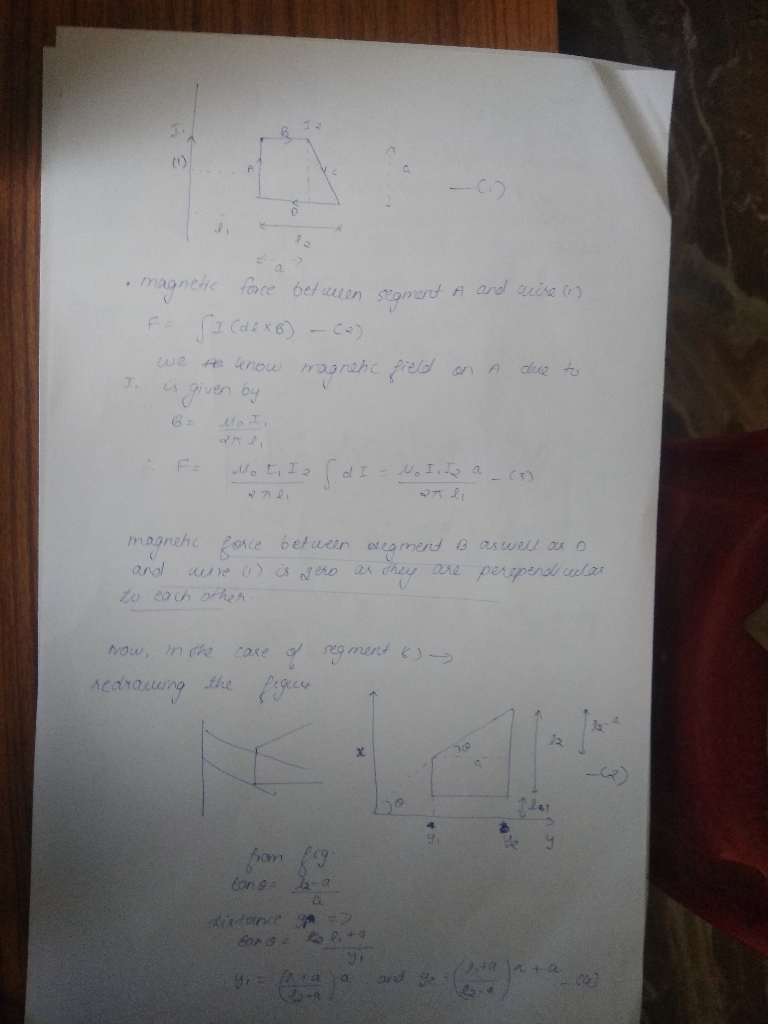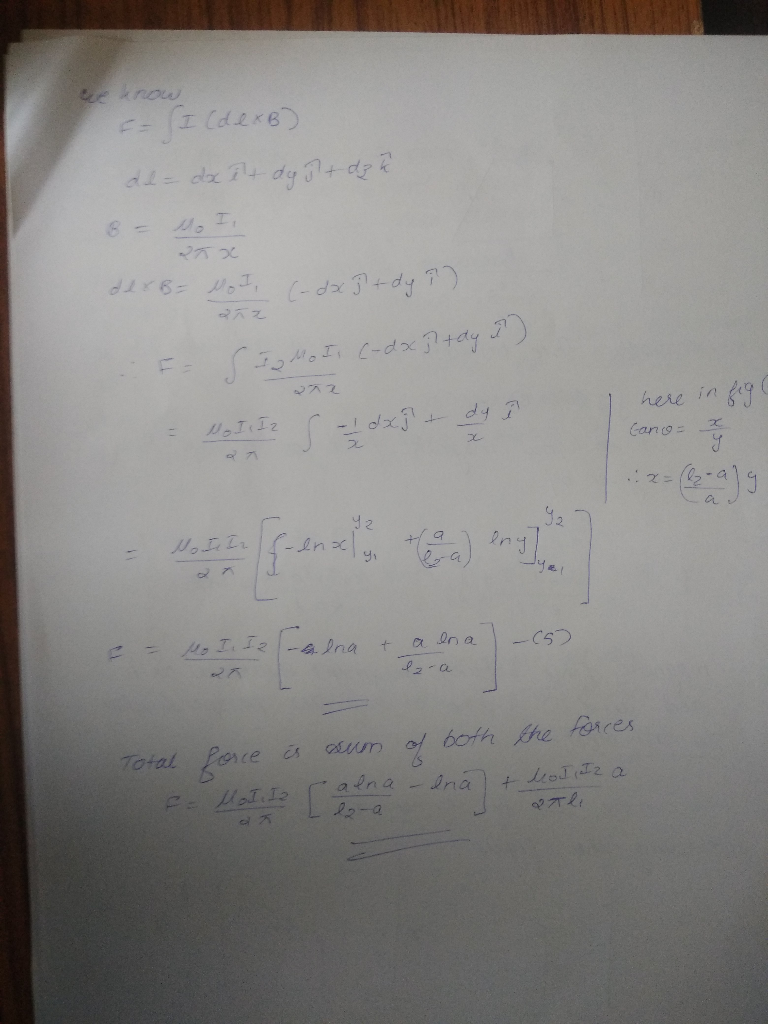# Q1) [50 points] Infinitely long wire is placed onto y-axis 7 and right angled trapezoid frame is placed onto xy-plane as in Figure 1. 11 and 12 currents flow into the wire and the frame respectively...Q1) [50 points] Infinitely long wire is placed onto y-axis 7 and right angled trapezoid frame is placed onto xy-plane as in Figure 1. 11 and 12 currents flow into the wire and the frame respectively with the shown directions. Figure 1 Calculate the net force acting on the frame. 2 I. .t 42##### Add Answer of: Q1) [50 points] Infinitely long wire is placed onto y-axis 7 and right angled trapezoid frame is placed onto xy-plane as in Figure 1. 11 and 12 currents flow into the wire and the frame respectively...
More Homework Help Questions Additional questions in this topic.